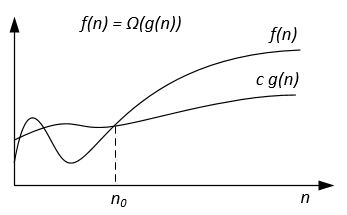# Big-Omega Notation

suggest change

Ω-notation is used for asymptotic lower bound.

# Formal definition

Let `f(n)` and `g(n)` be two functions defined on the set of the positive real numbers. We write `f(n) = Ω(g(n))` if there are positive constants `c` and `n0` such that:

`0 ≤ c g(n) ≤ f(n) for all n ≥ n0`.

# Notes

`f(n) = Ω(g(n))` means that `f(n)` grows asymptotically no slower than `g(n)`. Also we can say about `Ω(g(n))` when algorithm analysis is not enough for statement about `Θ(g(n))` or / and `O(g(n))`.

From the definitions of notations follows the theorem:

For two any functions `f(n)` and `g(n)` we have `f(n) = Ө(g(n))` if and only if `f(n) = O(g(n)) and f(n) = Ω(g(n))`.

Graphically Ω-notation may be represented as follows:For example lets we have `f(n) = 3n^2 + 5n - 4`. Then `f(n) = Ω(n^2)`. It is also correct `f(n) = Ω(n)`, or even `f(n) = Ω(1)`.

Another example to solve perfect matching algorithm : If the number of vertices is odd then output “No Perfect Matching” otherwise try all possible matchings.

We would like to say the algorithm requires exponential time but in fact you cannot prove a `Ω(n^2)` lower bound using the usual definition of `Ω` since the algorithm runs in linear time for n odd. We should instead define `f(n)=Ω(g(n))` by saying for some constant `c>0`, `f(n)≥ c g(n)` for infinitely many `n`. This gives a nice correspondence between upper and lower bounds: `f(n)=Ω(g(n))` iff `f(n) != o(g(n))`.

# References

Formal definition and theorem are taken from the book “Thomas H. Cormen, Charles E. Leiserson, Ronald L. Rivest, Clifford Stein. Introduction to Algorithms”.